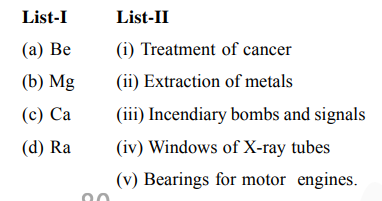# Match list-I with list-II :Question:

Match list-I with list-II :Choose the most appropriate answer the option given below:

1. a-iv, b-iii, c-i, d-ii

2. a-iv, b-iii, c-ii, d-i

3.  a-iii, b-iv, c-v, d-ii

4. a-iii, b-iv, c-ii, d-v

Correct Option: , 2

Solution:

(a) $\mathrm{Be} \rightarrow$ it is used in the Windows of X-ray tubes

(b) $\mathrm{Mg} \rightarrow$ it is used in the Incendiary bombs and signals

(c) $\mathrm{Ca} \rightarrow$ it is used in the Extraction of metals

(d) $\mathrm{Ra} \rightarrow$ it is used in the Treatment of cancer